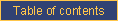homefeaturesexamplesDOCUMENTATIONblogpricingcontact ustechnical documentationCATI documentationcookbookrobotRandomly selecting a subset of possible answer categories

Problem

You want to show a random subset of possible answer categories to the respondent.

Solution

Use the select_random_combination function:

select_random_combination(among,number,exclusive,other_combination,other_combination,...)

where

• "among" is a positive integer stating the number of available options (e.g., the total number of magazines from which to choose);
• "number" is a positive integer indicating the size of the subset to select (e.g., 2 to select pairs of options);
• "exclusive" is 0 (zero) if a given option can be selected in more than one subset, or 1 (one) if it cannot;
• "other_combination,..." is a series of comma-delimited CallWeb question names (expressed as Perl variables, thus prefixed with a dollar sign) containing the names of all of the questions storing results of random selections of subsets from the same pool; this information is used to avoid picking the same combination twice and to avoid using the same integer twice if the selection is exclusive (see the parameter above).

Discussion

Sometimes, it is necessary to select a random subset of answer categories to be offered to the respondent. An example would be to randomly select two magazines and to ask the respondent to select the one they prefer. The special function select_random_combination is used for this purpose, along with the display of answer choices using category display conditions.

In the context of a CALCUL question, let's analyze the following call to this function:

Q1 = &select_random_combination(5,2,0,\$Q2,\$Q3)

This would place, in Q1 (which must have a maximum number of answers greater than 1 to accommodate pairs of selections or trios, etc.), a random selection of two integers between 1 and 5. The random selection will necessarily be different from the selections stored in Q2 and Q3 but it could include an integer already selected in Q2 or Q3.

Q1 = &select_random_combination(9,3,1,\$Q2,\$Q3)

This would place, in Q1, a random selection of three integers between 1 and 9. The random selection will necessarily be different from the selections stored in Q2 and Q3 and it cannot include an integer already selected in Q2 or Q3.

It is usually preferable to calculate these random selections only once so that, if the respondent backtracks in the questionnaire, he/she doesn't get assigned a different random selection. The following code achieves this:

Q1 = \$Q1 ? \$Q1 : &select_random_combination(9,3,1,\$Q2,\$Q3)

If it is not possible to find a combination because of the constraints imposed by the programmer, the function returns "---". This would happen:

• when trying to select more subsets than can be returned by a given situation (e.g., a fourth pair of integers between 1 and 3);
• in exclusive mode, when trying to select a new subset when other subsets leave fewer integers available than required to build a subset.

The result from this function can be used in another question which will display answer categories based on the calculated question. For example:

[Q1.EQ.1]
Choice no. 1
[Q1.EQ.2]
Choice no. 2
[Q1.EQ.3]
Choice no. 3
[Q1.EQ.4]
Choice no. 4

will display only the choices that were randomly selected in the Q1 subset.e-mail technical support!